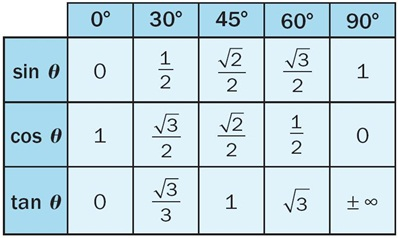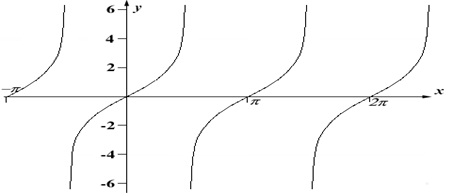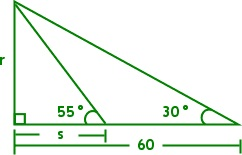×#### Thank you for registering.

One of our academic counsellors will contact you within 1 working day.

Click to Chat

1800-1023-196

+91-120-4616500

CART 0

• 0

MY CART (5)

Use Coupon: CART20 and get 20% off on all online Study Material

ITEM
DETAILS
MRP
DISCOUNT
FINAL PRICE
Total Price: Rs.

There are no items in this cart.
Continue Shopping```IIT JEE TrigonometryTrigonometry is a vital constituent of Mathematics in IIT JEE examination. Trigonometric functions and trigonometry ratios are some of the most imperative areas of trigonometry. Since the IIT JEE exam asks a good amount of questions on this topic, so getting the knack of the basic trigonometry can surely help an IIT JEE aspirant to smooth his way through the exam.

Trigonometry is the branch of Mathematics that deals with triangles and the relationships between the sides and angles. The trigonometry functions are universal in parts of pure mathematics and applied mathematics which also lay the groundwork for many branches of science and technology. The IIT JEE Trigonometry problems range from the trigonometry basics to the applications of trigonometry.

Some of the trigonometry questions are simply based on trigonometry formulae and are quite easy to crack while others may demand some trigonometry tricks. Thus IIT JEE trigonometry syllabus is a perfect blend of questions of all levels

Given below is the trigonometry table that illustrates the values of the functions at different angles:It would be an added advantage if the aspirants could memorize all above trigonometry formulas but if not; they must at least grasp the major ones like:The graphs also constitute a vital component in Trigonometry. A student who is well versed with the graphs of the major functions is able to tackle questions with ease. Some of the fundamental graphs are sketched below:Enlisted below are some of the prime heads that come under trigonometry and are covered in the coming sections:

Multiple and Sub-multiple Angles

Trigonometric Equations

Inverse circular Functions

Trigonometric Inequality

Trigonometric Functions

Trigonometric Identities and Equations

Illustration 1: Find the angles and sides indicated by the letters in the diagram. Give each answer correct to the nearest whole number.Solution:

Since in general, it is simpler to use the value of 30°, so we will have to consider the triangle with 30°.

Here, r/60 = tan 30°.

Hence, r = 60tan (30°) = 34.64101615...

So answer correct to the nearest whole number is r= 35.
Now, that we have obtained the value of r, we use the first triangle to evaluate s.

r/s = tan(55°)

so, 35/s = tan(55°)

so, 35/tan(55°) = s = 24.50726384...

r = 35, s = 25

Illustration 2: Evaluate the value of (1 + cos π/8) (1 + cos 3π/8) (1 + cos 5π/8) (1 + cos 7π/8).

Solution: The given expression is

(1 + cos π/8) (1 + cos 3π/8) (1 + cos 5π/8) (1 + cos 7π/8)

= (1 + cos π/8) (1 + cos 3π/8) (1 - cos 3π/8) (1 - cos π/8)

= (1 – cos 2π/8) (1 - cos 23π/8)

= ¼ [2 sin π/8 sin 3π/8]2

= ¼ [2 sin π/8 cos π/8]2

= ¼ [sin π/4]2

= 1/4. 1/2 = 1/8

Illustration 3: If cos (α – β) = 1 and cos (α + β) = 1/e, where α, β ∈ [-π, π], then the values of α and β satisfying both the equations is/are

Solution:  It is given in the question that cos (α – β) = 1 and cos (α + β) = 1/e, where α, β ∈ [-π, π].

Now, cos (α – β) = 1

α – β = 0, 2π, -2π

Hence, α – β = 0 (since α, β ∈ [-π, π])

So, α = β

Hence, cos 2α = 1/e

So, the number of solutions of above will be number of points of intersection of the curves y = cos 2α and y = 1/e, where α, β ∈ [-π, π]

It is quite clear that there are four solutions corresponding to four points of intersection P1, P2, P3 and P4.

Illustration 4: If k = sin (π/18) sin (5π/18) sin (7π/18), then what is the numerical value of k?

Solution: The value of k is given to be k = sin (π/18) sin (5π/18) sin (7π/18).

Hence, k = sin 10° sin 50° sin 70°

= sin 10° sin (60° - 10°). sin (60° + 10°)

= sin 10° [sin 260° - sin 210°]

= sin 10° [(√3/2)2 - sin 210°]

= sin 10° [3/4 - sin 210°]

= 1/4 [3sin 10° - 4sin 310°]

= 1/4 x sin (3 x 10)   (since, sin 3θ = 3sin θ- 4sin 3 θ)

= 1/4 sin 30° = 1/8

Hence, the numerical value of k is 1/8.

AskIITians offers you all IIT study material, question bank, mock test papers or free test series and that too in perfect alignment with the IIT JEE format. The study material covers all the major examples including those of the past year papers.

Look into the Revision Notes on Trigonometry for a quick revision.

Various Recommended Books of Mathematics are just a click away.

To read more, Buy study materials of Inverse Trigonometric Functions comprising study notes, revision notes, video lectures, previous year solved questions etc. Also browse for more study materials on Mathematics here.
```### Course Features

• 731 Video Lectures
• Revision Notes
• Previous Year Papers
• Mind Map
• Study Planner
• NCERT Solutions
• Discussion Forum
• Test paper with Video Solution